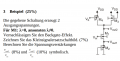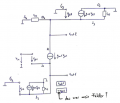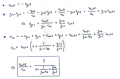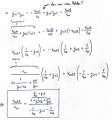# Small signal analysis of MOSFET circuit

#### marcell46

Joined Mar 3, 2021
2
The other day I got the following exercise on one of my exams:For those who don't speak German, for transistor 1 lambda is 0, but not for the others. The backgate-effekt should be neglected alltogether.

I drew the following small signal equivalent circuit (ignore the black texts for now):This should be fine, I got the whole 7% for that part of the exercise.

Now, my question is the following: are my calculations correct here? :I don't see where I am wrong. I got 2% and 2% instead of the 8% and 10%, so 4% out of the 18%. But as far as I see, I only had two problems in my calculations, I accidentally took vgs2 with positive sign (bad mistake, I know, I drew it correctly, but still got the wrong sign), and in the current calculations I should have added the gm3*vgs3 current because of the direction, but I subtracted it by mistake (again, the direction was drawn correctly, yet I made another mistake).

In these calculations that I posted, I recognized these mistakes and corrected them. The question is, is there something else that I am not getting here? Still some currents in the wrong direction? Some wrong calculation? I did not get any solutions, I only got a comment, saying: "current flows towards source, and as for PMOS, per definition negative". And don't really get what this means, I wrote to the tutor but it will take some time for me to get an answer and even if I do it probably won't explain my mistakes properly.

The mistakes that I found are bad mistakes, I get it, but I don't think that they are worth 77.8% of the exercise, so there must be something else. Also, this comment must mean something that I don't yet understand.

All help and explanation is appreciated!

#### Jony130

Joined Feb 17, 2009
5,333
Your gain equation for Vout 1 is wrong. How can this gain be larger than 1? If I write the gain expression by inspection I've got this

$\Large \frac{V_{Out1}}{V_{IN}} = \frac{\frac{1}{g_{m2}}||ro2}{\frac{1}{g_{m1}}+\frac{1}{g_{m2}}||ro2}$

And for me, the gain expression for Vourt2 should have looked something like this (again I write it by inspection):

$\Large \frac{V_{Out2}}{V_{IN}} =- \frac{\frac{1}{g_{m3}}||r_{o3}||r_{o4} }{ \frac{1}{g_{m1}} +\frac{1}{g_{m2}}||r_{o2} }$

Last edited:

#### marcell46

Joined Mar 3, 2021
2
Your gain equation for Vout 1 is wrong. How can this gain be larger than 1? If I write the gain expression by inspection I've got this

$\Large \frac{V_{Out1}}{V_{IN}} = \frac{\frac{1}{g_{m2}}||ro2}{\frac{1}{g_{m1}}+\frac{1}{g_{m2}}||ro2}$

And for me, the gain expression for Vourt2 should have looked something like this (again I write it by inspection):

$\Large \frac{V_{Out2}}{V_{IN}} =- \frac{\frac{1}{g_{m3}}||r_{o3}||r_{o4} }{ \frac{1}{g_{m1}} +\frac{1}{g_{m2}}||r_{o2} }$
First of all, thank You for your reply. But if I am not mistaken, you result for Vout1 is the same as mine if I write reorder it. But nonetheless, all I did was to write equations based on Kirchhoff's laws of current and voltage and then do the mathematical calculations. I am sure that it is more elegant (and after some practice probably easier) to write the solution based on "inspection". But as for me, I need structurally sound and always working solution. And as fas as I can tell, it goes like this: I know the small signal equivalent circuit for MOSFET, I draw the correct small signal eqivalent circuit for the whole circuit, I apply Kirchhoff's laws to write the correct equations and then use math to solve the equation system. This should be the fool-proof mechanism. But it is promising that we got to the same solutionor my math is really off again xd

#### Jony130

Joined Feb 17, 2009
5,333
Yes, you are right I made an error when typing your equation. I do apologize for the confusion. And "inspection" I meant, that I wrote the equations just by looking onto the circuit schematic.